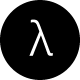# UVa 10706. Number Sequence with F#

This post describes an algorithm to solve UVa 10706: Number Sequence problem from UVa OJ. Before outlining the algorithm, we first give an overview of this problem in the next section.

# Interpretation

This is a straight-forward problem, which however, requires careful introspection. Our objective is to write a program that compute thevalue of a sequence, which is comprised of the number groups. Eachconsists of positive integerswritten one after another and thus, the firstdigits ofare —

`11212312341234512345612345671234567812345678912345678910123456789101112345678910`

It is imperative to note that, the value ofhas the following range. Realize that, the maximum value ofis indeed `Int32.MaxValue`. In the provided test cases, the values ofare stated. We simply have to compute; therefore, we are required to write a functionthat has following signature:.  That is —

```f: 10 --> S. = 4
f: 8  --> S.  = 2
f: 3  --> S.  = 2```

Note also that the maximum number of test cases is 25. Question for the reader: What is the maximum possible value offor the specified sequence, which is constituted of?

We hope that by this time, it is clear where we are headed towards. In next section, we outline an algorithm to solve this problem. But, before moving further, we would like to recommend you to try to solve it yourselves first and then, read the rest of the post. By the way, we believe that the solution that we have presented next can be further optimized (or, even a better solution can be derived). We highly welcome your feedback regarding this.

# Algorithm

Recall that,is constituted of number groups. In order to identify the digit located at theposition of, we first determine the number groupthatdigit is associated with and then, we figure out thedigit from.

To do so, we first compute the length of each number group. Consider the number group till`112123123412345123456…12345678910`

As mentioned, this sequence is basically constituted ofas shown below (with their respective lengths).

```1                    --> 1
1 2                  --> 2
1 2 3                --> 3
1 2 3 4              --> 4
1 2 3 4 5            --> 5
1 2 3 4 5 6          --> 6
1 2 3 4 5 6 7        --> 7
1 2 3 4 5 6 7 8      --> 8
1 2 3 4 5 6 7 8 9    --> 9
1 2 3 4 5 6 7 8 9 10 --> 11```

It implies that the length ofcan be computed from the length ofas shown below.Using Eq.1, we calculate the cumulative sum of each number group as follows.Why do we calculate cumulative sum? The purpose in this is to be able to simply run a binary search to determine which number group thedigit belongs to intime. For example, consider `i=40`.

```1                    --> 1
1 2                  --> 2
1 2 3                --> 6
1 2 3 4              --> 10
1 2 3 4 5            --> 15
1 2 3 4 5 6          --> 21
1 2 3 4 5 6 7        --> 28
1 2 3 4 5 6 7 8      --> 36
1 2 3 4 5 6 7 8 9    --> 45
1 2 3 4 5 6 7 8 9 10 --> 56```

Using binary search, we can find out thatcontains thedigit. Then, using a linear search, we can simply derive the first four digits ofto eventually find out the corresponding digit, `4`.Similarly, for `i=55`, we can figure out that the digit is indeed `1`.

# Implementation

Next, we outline a F# implementation of the stated algorithm. We start with computing the cumulative sums of the lengths as follows.

 let arr = [1..31267] // max K |> getNumLengths |> cumulativeSumofLengths |> List.toArray
view raw Uva10706.fsx hosted with ❤ by GitHub

Then, for each `i`, we perform a binary search to locate.

 (* * Performs a binary search on the cumulative sums of lengths. * and return a tuple as (minBound, maxBound) * : arr: int[] -> n: int -> (int,int) *) let getBounds a (n:int): int*int = let binarySearch (arr:int[]) (n:int) : (int*int) = let rec binarySearch' (arr:int[]) (n:int) (low:int) (high:int) = if arr.[high] < n then (high, System.Int32.MaxValue) else if high - low <= 1 then if arr.[low] = n then (low, low) else (low,high) else let mid = (high + low)/2 match arr.[mid],n with | (a,b) when a <= b-> binarySearch' arr n mid high | _ -> binarySearch' arr n low mid binarySearch' arr n 0 (arr.Length-1) binarySearch a n
view raw Uva10706.fsx hosted with ❤ by GitHub

Lastly, we perform a linear search to figure out the `ith` digit from.

 let getDigitAtIndex (relLength:int) (index:int): int = let rec getIntAtIndex' (relLength:int) (startNum:int)(endNum:int):int = if startNum <= endNum then let str = startNum.ToString() match relLength - str.Length with | diff when diff <= 0 -> System.Int32.Parse(str.[relLength-1].ToString()) | diff -> getIntAtIndex' (relLength - str.Length) (startNum+1) endNum else -1 getIntAtIndex' relLength 1 index
view raw Uva10706.fsx hosted with ❤ by GitHub

Complexity.where  the value ofis bounded above by.

For complete implementation of this algorithm, please visit this gist. More so, to play further with this implementation, we have created a IDEONE page with sample input and corresponding output. Please leave a comment if there is any question or suggestion. Thanks!

## 5 thoughts on “UVa 10706. Number Sequence with F#”

1.ilevis says:

good job.
Thanks :v

2.Kaidul Islam Sazal says:

Thanks for nice explanation. I have solved in C++ :) Want more explanatory posts.

3.Verb says:

Great explanation but I can’t see your equations, so I’m still a bit lost :-(

1.adilakhter says:

… will check if I can fix it.